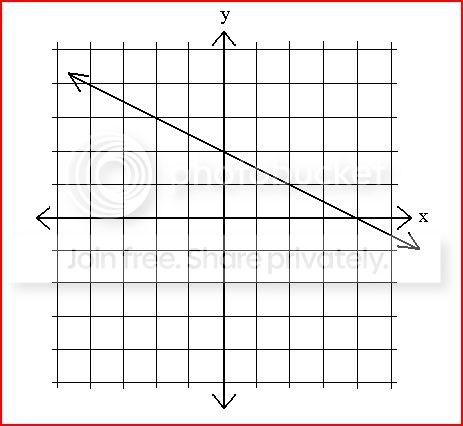Pre-algebra Summer Quiz 2

10 Questions | Total Attempts: 247SettingsThis quiz covers material that we reviewed during the first three lessons of your summer Pre-Algebra online course.  Good luck!

• 1.
What is the slope of this line?
• A.

- 1/2

• B.

2

• C.

-2

• D.

1/4

• 2.
Which point is a solution to the line 2y = -8 + 3x?
• A.

(0,0)

• B.

(1,4)

• C.

(3,2)

• D.

(4,2)

• 3.
Solve for x:
• A.

1

• B.

2

• C.

-1

• D.

-2

• 4.
The ordered pair (3,0) is found:
• A.

On the y-axis

• B.

On the x-axis

• C.

• D.

At the origin

• 5.
Solve the equation:
• A.

-31

• B.

-85.4

• C.

-50

• D.

-35

• 6.
A line passes through the points (1,-5) and (-3,7).What is its slope?
• A.

3

• B.

-3

• C.

1/3

• D.

-1/3

• 7.
What is the equation of the VERTICAL line passing through the point (-2,5)?
• A.

X = -2

• B.

Y = -2

• C.

X = 5

• D.

Y = 5

• 8.
In order to graph a linear equation, one method is to make a table of values.Which of the following points would appear in a table of values for the line?
• A.

(1,-1)

• B.

(2,0)

• C.

(3,3)

• D.

(-3,-9)

• E.

(-1,5)

• 9.
Which option contains only points that lie on the line represented by ?
• A.

(0,-6) and (9,6)

• B.

(0,6) and (12,2)

• C.

(-3,-8) and (6,-2)

• D.

(-3,-4) and (3,-2)

Related TopicsBack to top